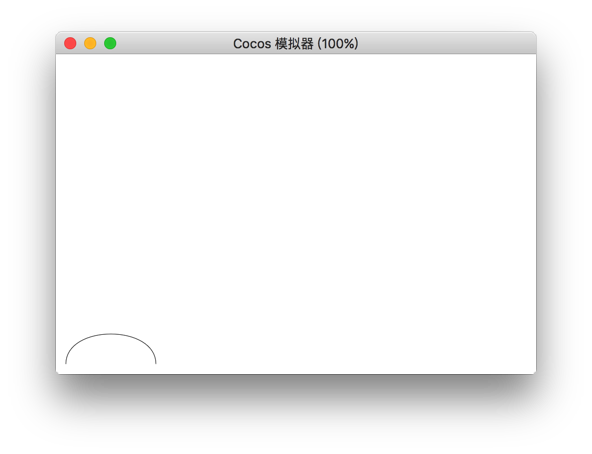# BezierCurveTo

The `bezierCurveTo()` method adds a point to the current path by using the specified control point that represents the cubic bezier curves. Tip: Cubic Bezier curves require three control points. The first two points are for the control points in the cubic Bezier calculation, and the third point is the end point of the curve. The starting point of the curve is the last point in the current path.

Parameter description
cp1x The first Bezier control point of the x coordinate
cp1y The y coordinate of the first Bezier control point
cp2x x coordinate of the second Bezier control point
cp2y The second coordinate of the second Bezier control point
x The x coordinate of the end point
y The y coordinate of the end point

## Example

``````var ctx = node.getComponent (cc.Graphics);
ctx.moveTo (20,20);
ctx.bezierCurveTo (20,100,200,100,200,20);
ctx.stroke ();
``````Return to Graphics Component Reference